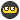## Recommended Posts

So it looks like my compass problem is nearing a solution(other post), but a new function I have added is not behaving as expected. I tried to test a similar function seperately to find the same behaviour.

Global variables can be altered from anywhere, but Function variables are destroyed the moment the function ends. I use global variables within functions all the time, I just don't know why this one is affected. In this function the global variable is altered within the function. Surely after the function is called the modified values should show up but that's not the case. Even after the function, which modifies these global variables, these variables are still the same as before the function was called.

Below is a test script. It changes both test_nums from a value of 0 to 50. The msgbox should return the modified value since it's after the function call, but it instead returns the non-modified value. This leads me to conclude these global variables are acting as function variables and are destroyed the moment the function ends.

```\$test_num1 = 0;
\$test_num2 = 0;

calc(\$test_num1,\$test_num2);

msgbox(0, "test", \$test_num1&","&\$test_num2);

Func calc(\$test_num1,\$test_num2);
If \$test_num1 = 0 Then
\$test_num1 = 50;
EndIf
If \$test_num2 = 0 Then
\$test_num2 = 50;
EndIf
EndFunc```

##### Share on other sites

Use a different variable name in the function call than in the global variable. Also, use the byref keyword to allow the function to change the global variable.

```\$test_num1 = 0;
\$test_num2 = 0;

calc(\$test_num1,\$test_num2);

msgbox(0, "test", \$test_num1&","&\$test_num2);

Func calc(Byref \$x,Byref \$y);
If \$x = 0 Then
\$x = 50;
EndIf
If \$y = 0 Then
\$y = 50;
EndIf
EndFunc```

here, \$x only references \$test_num1 when passed to the function by calc(\$test_num1,\$test_num2);

Edited by this-is-me

Who else would I be?

##### Share on other sites

Or try this:

Your var isn't 'global' in the function.

```\$test_num1 = 0;
\$test_num2 = 0;
calc(\$test_num1,\$test_num2);
msgbox(0, "test", \$test_num1&","&\$test_num2);

Func calc(\$test_num1,\$test_num2);
If \$test_num1 = 0 Then
global \$test_num1 = 50;
EndIf
If \$test_num2 = 0 Then
global \$test_num2 = 50;
EndIf
EndFunc```

Tested...##### Share on other sites

Yet another example of why using "MustDeclareVars" should be on by default... Forcing a keyword to declare the scope would of eliminated this entirely.

##### Share on other sites

Ah now I get it.

You learn something new everyday. Actually that makes 2 things today ^^.

Thanks again guys.

##### Share on other sites

Another thing to do is to declare any variable you use in a function as Local. This does not actually apply this time, as the function arguments are automatically local. Take a look at Local in the help file.

David Nuttall
Nuttall Computer Consulting

An Aquarius born during the Age of Aquarius

AutoIt allows me to re-invent the wheel so much faster.

I'm off to write a wizard, a wonderful wizard of odd...

## Create an account

Register a new account

• ### Recently Browsing   0 members

×

• Wiki

• Back

• #### Beta

• Git
• FAQ
• Our Picks
×
• Create New...